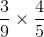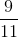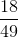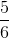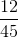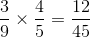# SSAT Middle Level Math : How to multiply fractions

## Example Questions

### Example Question #5 : Operations With Fractions And Whole Numbers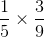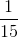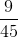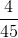Explanation:

When we multiply fractions, we multiply the numerator by the numerator and the denominator by the denominator.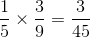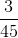can be reduced by dividing both sides by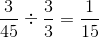### Example Question #61 : How To Multiply Fractions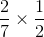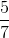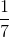Explanation:

When we multiply fractions, we multiply the numerator by the numerator and the denominator by the denominator.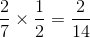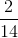can be reduced by dividing both sides by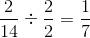### Example Question #1 : Operations With Fractions And Whole Numbers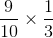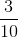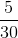Explanation:

When we multiply fractions, we multiply the numerator by the numerator and the denominator by the denominator.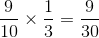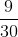can be reduced by dividing both sides by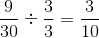### Example Question #61 : How To Multiply Fractions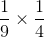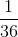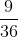Explanation:

When we multiply fractions, we multiply the numerator by the numerator and the denominator by the denominator.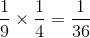### Example Question #1 : Multiply A Fraction Or Whole Number By A Fraction: Ccss.Math.Content.5.Nf.B.4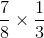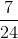Explanation:

When we multiply fractions, we multiply the numerator by the numerator and the denominator by the denominator.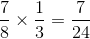### Example Question #61 : How To Multiply Fractions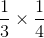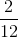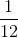Explanation:

When we multiply fractions, we multiply the numerator by the numerator and the denominator by the denominator.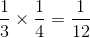### Example Question #231 : Fractions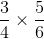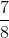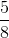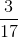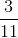Explanation:

When we multiply fractions, we multiply the numerator by the numerator and the denominator by the denominator.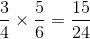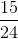can be reduced by dividing both sides by.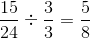### Example Question #231 : Fractions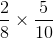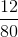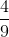Explanation:

When we multiply fractions, we multiply the numerator by the numerator and the denominator by the denominator.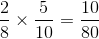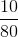can be reduced by dividing each side by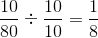### Example Question #71 : How To Multiply Fractions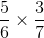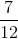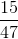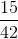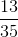Explanation:

When we multiply fractions, we multiply the numerator by the numerator and the denominator by the denominator.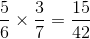### Example Question #11 : Multiply A Fraction Or Whole Number By A Fraction: Ccss.Math.Content.5.Nf.B.4